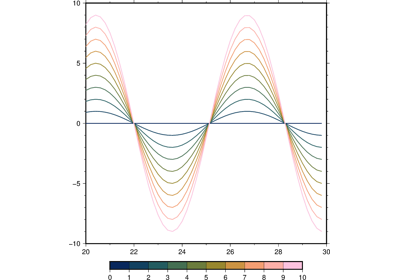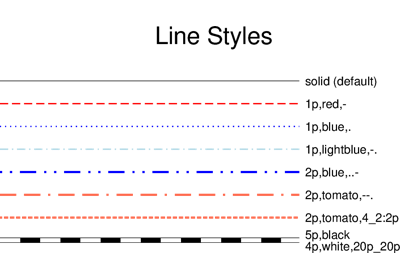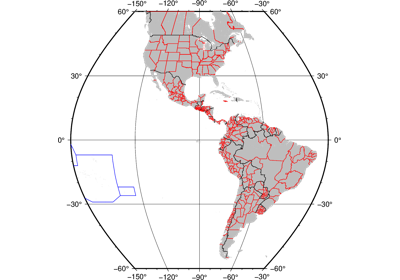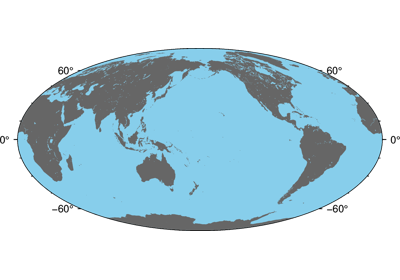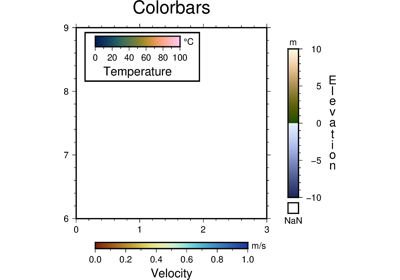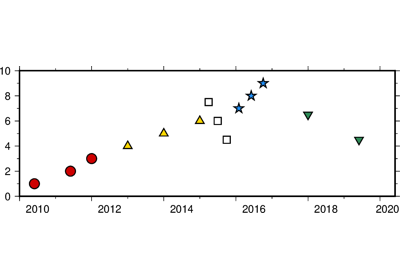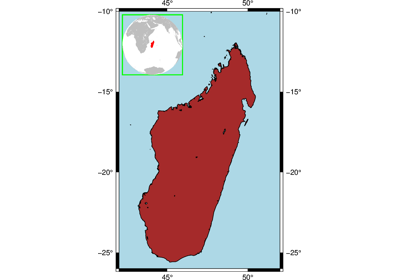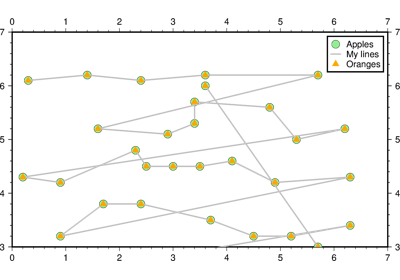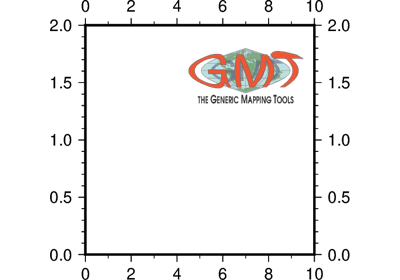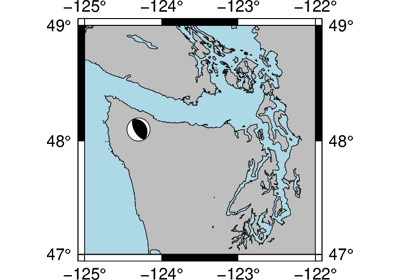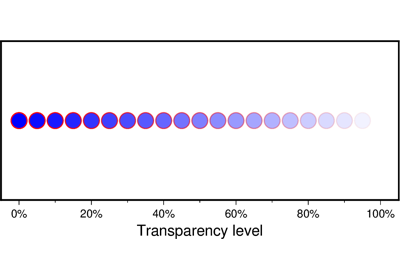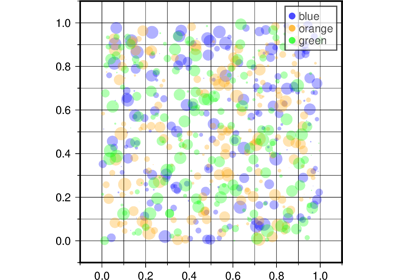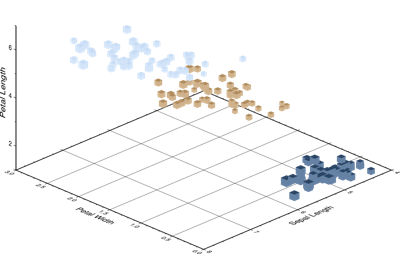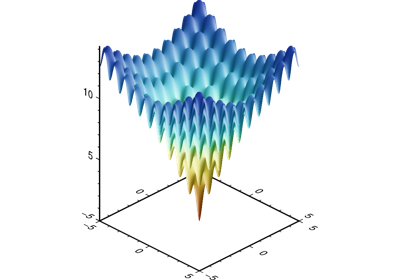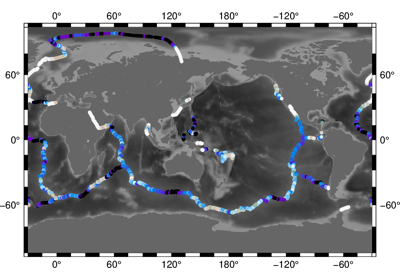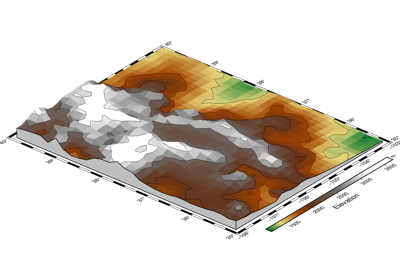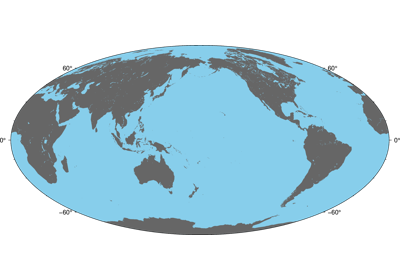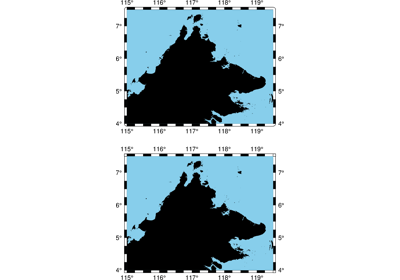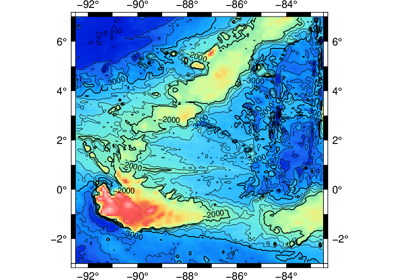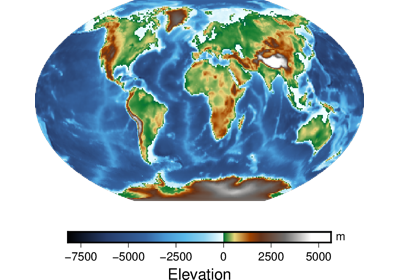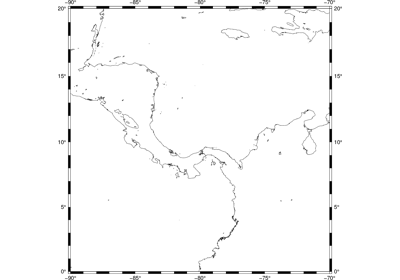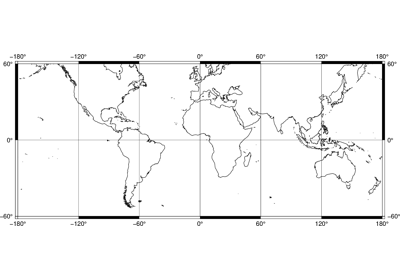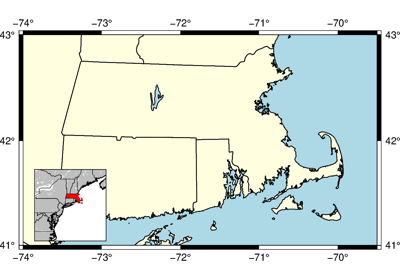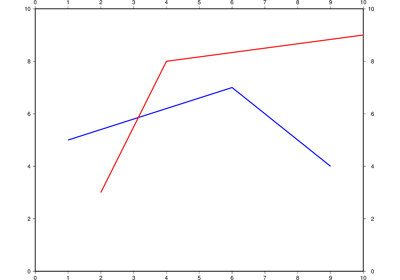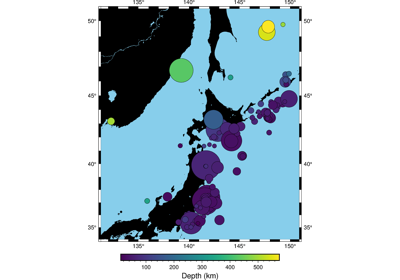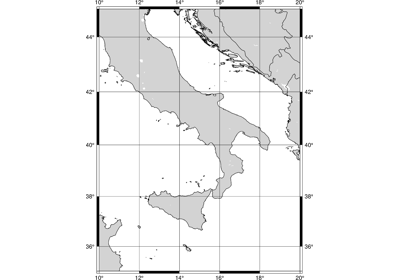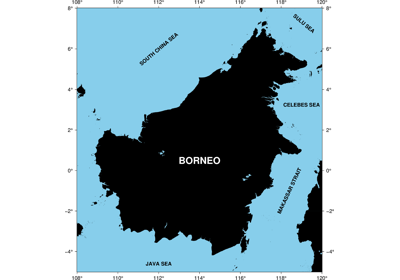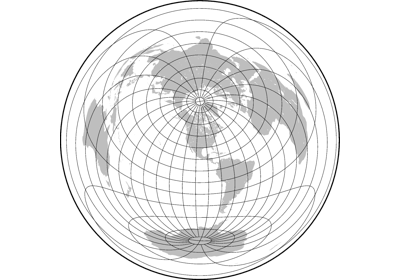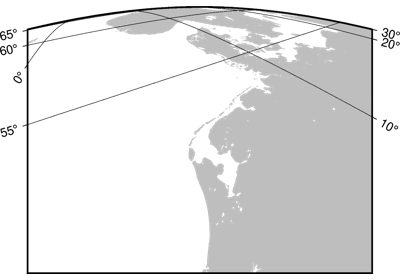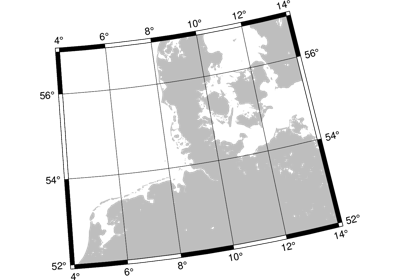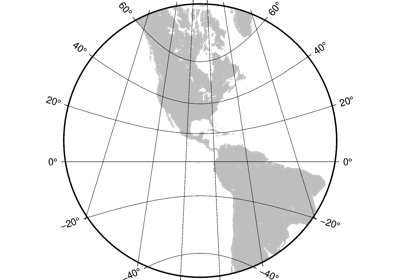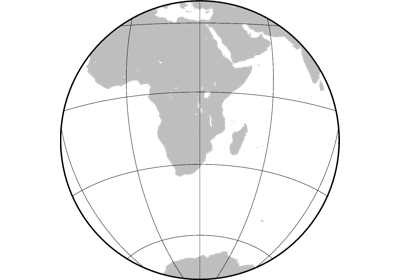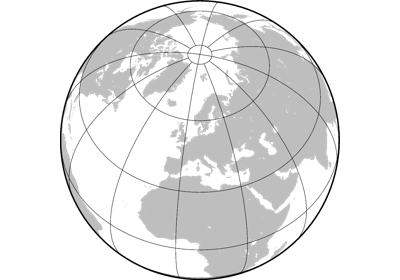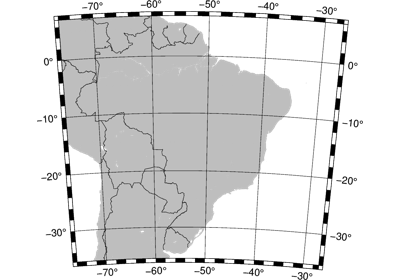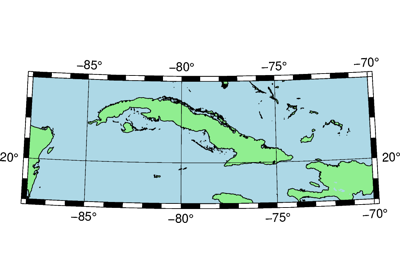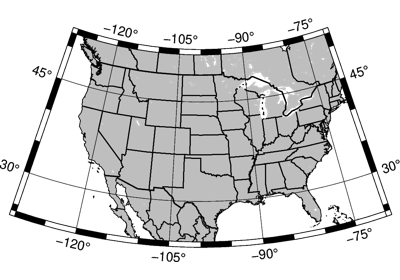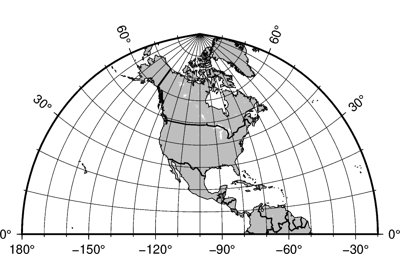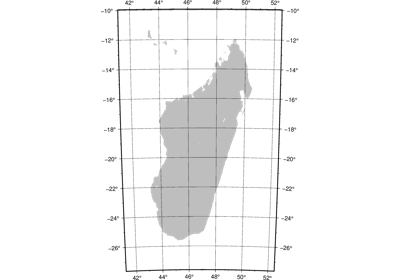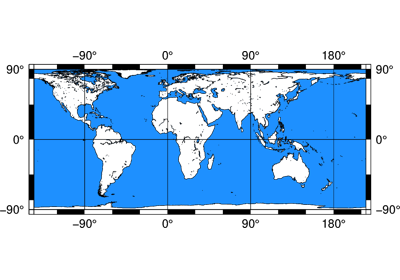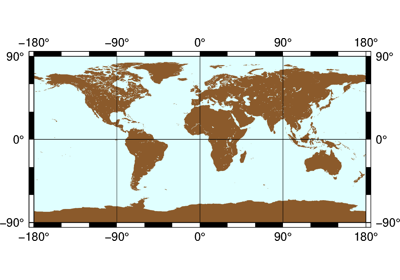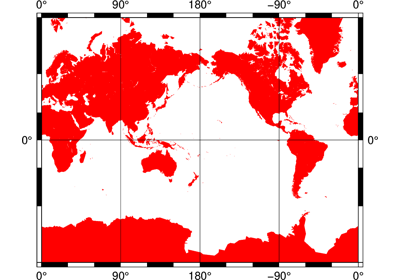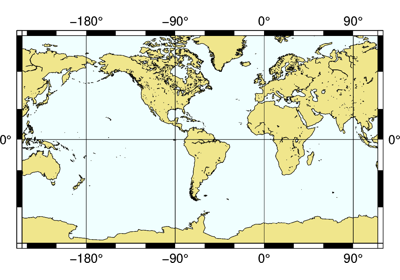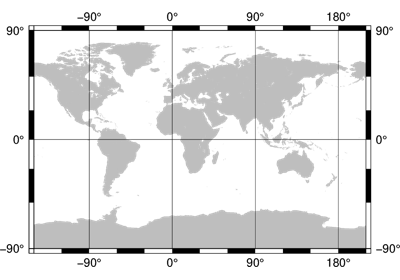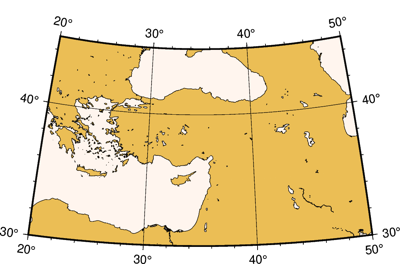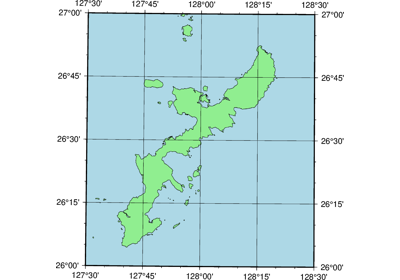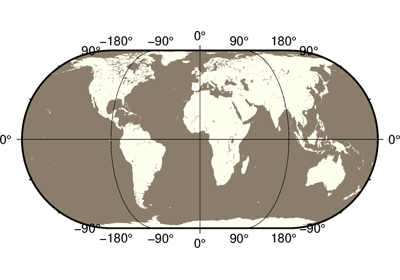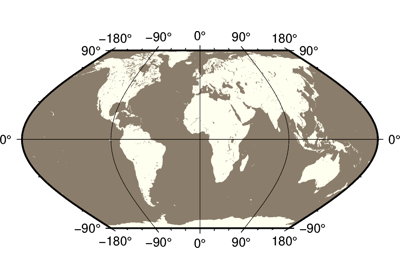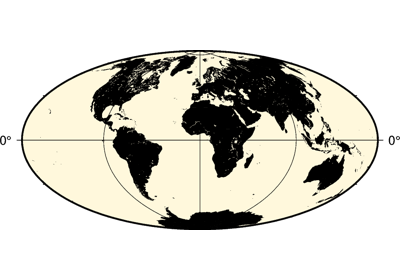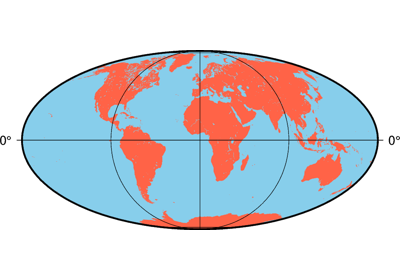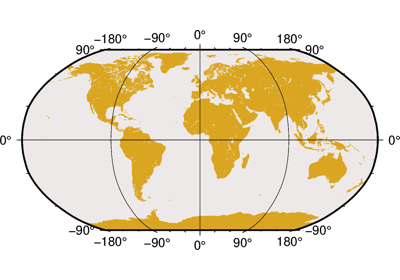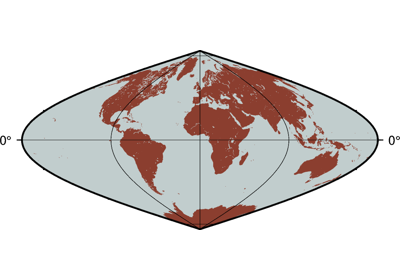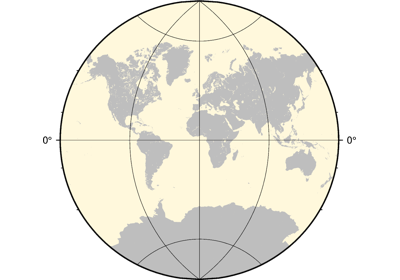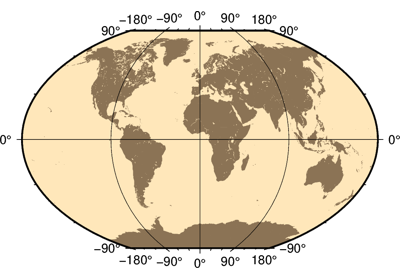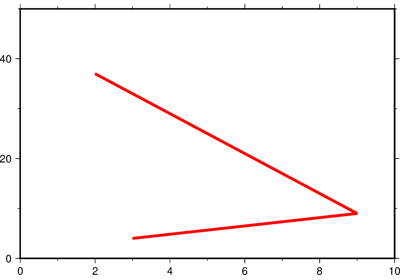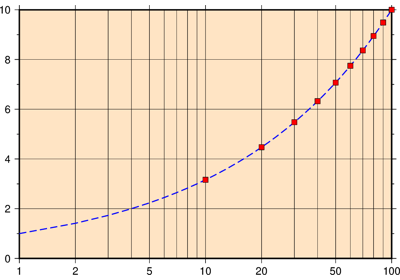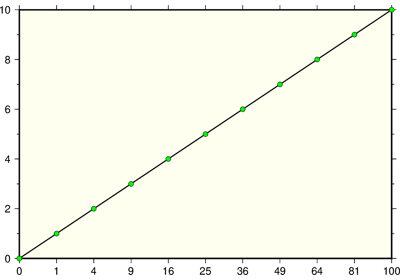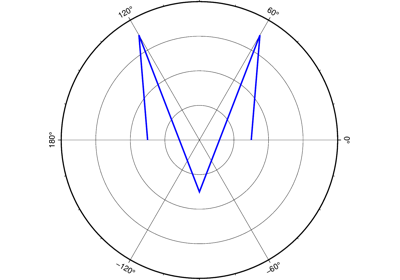# pygmt.Figure¶

class `pygmt.``Figure`[source]

A GMT figure to handle all plotting.

Use the plotting methods of this class to add elements to the figure. You can preview the figure using `pygmt.Figure.show` and save the figure to a file using `pygmt.Figure.savefig`.

Unlike traditional GMT figures, no figure file is generated until you call `pygmt.Figure.savefig` or `pygmt.Figure.psconvert`.

Examples

```>>> fig = Figure()
>>> fig.basemap(region=[0, 360, -90, 90], projection="W7i", frame=True)
>>> fig.savefig("my-figure.png")
>>> # Make sure the figure file is generated and clean it up
>>> import os
>>> os.path.exists("my-figure.png")
True
>>> os.remove("my-figure.png")
```

The plot region can be specified through ISO country codes (for example, `'JP'` for Japan):

```>>> fig = Figure()
>>> fig.basemap(region="JP", projection="M3i", frame=True)
>>> # The fig.region attribute shows the WESN bounding box for the figure
>>> print(", ".join("{:.2f}".format(i) for i in fig.region))
122.94, 145.82, 20.53, 45.52
```

Methods Summary

 `Figure.basemap`(**kwargs) Plot base maps and frames for the figure. `Figure.coast`(**kwargs) Plot continents, shorelines, rivers, and borders on maps `Figure.colorbar`(**kwargs) Plot a gray or color scale-bar on maps. `Figure.contour`([x, y, z, data]) Contour table data by direct triangulation. `Figure.grdcontour`(grid, **kwargs) Convert grids or images to contours and plot them on maps. `Figure.grdimage`(grid, **kwargs) Project and plot grids or images. `Figure.grdview`(grid, **kwargs) Create 3-D perspective image or surface mesh from a grid. `Figure.image`(imagefile, **kwargs) Place images or EPS files on maps. `Figure.inset`(**kwargs) Create an inset figure to be placed within a larger figure. `Figure.legend`([spec, position, box]) Plot legends on maps. `Figure.logo`(**kwargs) Plot the GMT logo. `Figure.meca`(spec, scale[, longitude, …]) Plot focal mechanisms. `Figure.plot`([x, y, data, sizes, direction]) Plot lines, polygons, and symbols in 2-D. `Figure.plot3d`([x, y, z, data, sizes, direction]) Plot lines, polygons, and symbols in 3-D. `Figure.psconvert`(**kwargs) Convert [E]PS file(s) to other formats. `Figure.savefig`(fname[, transparent, crop, …]) Save the figure to a file. `Figure.set_panel`([panel]) Set the current subplot panel to plot on. `Figure.shift_origin`([xshift, yshift]) Shift plot origin in x and/or y directions. `Figure.show`([dpi, width, method]) Display a preview of the figure. `Figure.subplot`([nrows, ncols]) Create multi-panel subplot figures. `Figure.text`([textfiles, x, y, position, …]) Plot or typeset text strings of variable size, font type, and orientation.

## Examples using `pygmt.Figure`¶